SNAS470E October   2008  – November 2015

PRODUCTION DATA.

1. Features
2. Applications
3. Description
4. Revision History
5. Pin Configuration and Functions
6. Specifications
7. Detailed Description
1. 7.1 Overview
2. 7.2 Functional Block Diagram
3. 7.3 Feature Description
4. 7.4 Device Functional Modes
5. 7.5 Programming
6. 7.6 Register Maps
8. Application and Implementation
1. 8.1 Application Information
2. 8.2 Typical Application
9. Power Supply Recommendations
10. 10Layout
1. 10.1 Layout Guidelines
2. 10.2 Layout Example
11. 11Device and Documentation Support
12. 12Mechanical, Packaging, and Orderable Information

• PWP|14
• PWP|14

## 6 Specifications

### 6.1 Absolute Maximum Ratings

See (1)(2)(3)
MIN MAX UNIT
Supply voltage, continuous 6 V
Input voltage −0.3 VDD + 0.3 °C
Power dissipation(4) Internally Limited
Junction temperature 150 °C
Lead temperature (soldering 4 sec)(5) 260 °C
Storage temperature −65 150 °C
(1) Stresses beyond those listed under Absolute Maximum Ratings may cause permanent damage to the device. These are stress ratings only, which do not imply functional operation of the device at these or any other conditions beyond those indicated under Recommended Operating Conditions. Exposure to absolute-maximum-rated conditions for extended periods may affect device reliability.
(2) The Electrical Characteristics tables found in Specifications list ensured specifications under the listed Recommended Operating Conditions except as otherwise modified or specified by the Electrical Characteristics Test Conditions, Notes, or both. Typical specifications are estimations only and are not ensured.
(3) If Military/Aerospace specified devices are required, please contact the Texas Instruments Sales Office/Distributors for availability and specifications.
(4) θJA measured with a 4 layer JEDEC board.
(5) For detailed information on soldering plastic HTSSOP and LLP packages go to the TI Packaging site, ti.com/packaging.

### 6.2 ESD Ratings

VALUE UNIT
V(ESD) Electrostatic discharge Human-body model (HBM), per AEC Q100-002(1) 2500 V
Charged-device model (CDM), per AEC Q100-011 300
(1) AEC Q100-002 indicates that HBM stressing shall be in accordance with the ANSI/ESDA/JEDEC JS-001 specification.

### 6.3 Recommended Operating Conditions

MIN MAX UNIT
Temperature TMIN ≤ TA ≤ TMAX −40 105 °C
Supply voltage VDDand PVDD 3 5.5 V
I2C Supply voltage I2CVDD 1.8 5.5 V
I2CVDD VDD V

### 6.4 Thermal Information

THERMAL METRIC(1) LM48100Q-Q1 UNIT
PWP (HTSSOP)
14 PINS
RθJA Junction-to-ambient thermal resistance 37.8 °C/W
RθJC(top) Junction-to-case (top) thermal resistance 5.2 °C/W
RθJB Junction-to-board thermal resistance °C/W
ψJT Junction-to-top characterization parameter °C/W
ψJB Junction-to-board characterization parameter °C/W
RθJC(bot) Junction-to-case (bottom) thermal resistance °C/W

### 6.5 Electrical Characteristics for VDD = 5 V

Programmable Gain = 0 dB, RL = 8 Ω, f = 1 kHz, unless otherwise specified. Limits apply for TA = 25°C, unless otherwise specified.
PARAMETER TEST CONDITIONS MIN(1) TYP(2) MAX UNIT
IDD Quiescent Power Supply Current VIN = 0 V, Both channels active RL = 8 Ω 4.4 9 mA
RL = ∞ 4.2 6
IDD Diagnostic Mode Quiescent Power Supply Current Diagnostic Mode Enabled, RL = ∞ 12.5 14.5 mA
ISD Shutdown Current Shutdown Enabled 0.01 1 µA
VOS Differential Output Offset Voltage VIN = 0 V, RL = 8 Ω 8.8 50 mV
TWU Wake-Up Time Time from shutdown to audio available 11.6 50 ms
AV Gain Minimum Gain Setting –55 –54 –53 dB
Maximum Gain Setting 17 18 19
Mute Mute Attenuation –80 –77 dB
RIN Input Resistance AV = 18 dB 11.5 12.5 13.5
AV = –54 dB 98 110 120
PO Output Power RL = 8 Ω, f = 1 kHz THD+N = 10% 1.6 W
THD+N = 1% 1.05 1.3
THD+N Total Harmonic Distortion + Noise PO = 850 mW, f = 1 kHz, RL = 8 Ω 0.04%
PSRR Power Supply Rejection Ratio VRIPPLE = 200 mVP-P Sine, Inputs AC GND, CIN_= 1 μF, Input Referred, CBIAS = 2.2 μF f = 217 Hz 66 79 dB
f = 1 kHz 74
SNR Signal-to-Noise-Ratio POUT = 450 mW, f = 1 kHz 104 dB
OS Output Noise AV = 0 dB, A-weighted Filter 12 μV
IOUT(FAULT) FAULT Output Current FAULT = 0, VOUT(FAULT)= 0.4 V 3 mA
RFAULT Output to Supply Short Circuit Detection Threshold Short between either OUTA to VDD or GND, or OUTB to VDD or GND Short Circuit 3
Open Circuit 7.5
RFAULT Output to Supply Short Circuit Detection Threshold Short between both OUTA and
OUTB to VDD or GND
Short Circuit 6
Open Circuit 15
ROPEN Open Circuit Detection Threshold Open circuit between OUTA and OUTB 100 200 Ω
RSHT Output to Output Short Circuit Detection Threshold Short circuit between OUTA and OUTB 2 6 Ω
ISHTCKT Short Circuit Current Limit 1.47 1.67 A
TSD Thermal Shutdown Threshold 170 °C
tDIAG Diagnostic Time 58 ms
(1) Datasheet min/max specification limits are specified by test or statistical analysis.
(2) Typical Values are given for TA = 25°C.

### 6.6 Electrical Characteristics for VDD = 5 V at Extended Temperature Limits

Programmable Gain = 0 dB, RL = 8 Ω, f = 1 kHz, unless otherwise specified. Limits apply for TA = –40°C to 105°C, unless otherwise specified.
PARAMETER TEST CONDITIONS MIN(1) TYP(2) MAX UNIT
IDD Quiescent Power Supply Current VIN = 0 V, Both channels active RL = 8 Ω 4.4 10.8 mA
RL = ∞ 4.2 7.9
IDD Diagnostic Mode Quiescent Power Supply Current Diagnostic Mode Enabled, RL = ∞ 12.5 mA
ISD Shutdown Current Shutdown Enabled 0.01 µA
VOS Differential Output Offset Voltage VIN = 0 V, RL = 8 Ω 8.8 75 mV
TWU Wake-Up Time Time from shutdown to audio available 11.6 ms
AV Gain Minimum Gain Setting –56 –54 –52 dB
Maximum Gain Setting 17 18 19
Mute Mute Attenuation –80 –74 dB
RIN Input Resistance AV = 18 dB 12.5
AV = –54 dB 89 110 130
PO Output Power RL = 8 Ω, f = 1 kHz THD+N = 10% 1.6 W
THD+N = 1% 0.96 1.3
THD+N Total Harmonic Distortion + Noise PO = 850 mW, f = 1 kHz, RL = 8 Ω 0.04%
PSRR Power Supply Rejection Ratio VRIPPLE = 200 mVP-P Sine, Inputs AC GND, CIN_= 1 μF, Input Referred, CBIAS = 2.2 μF f = 217 Hz 63 79 dB
f = 1 kHz 74
SNR Signal-to-Noise-Ratio POUT = 450 mW, f = 1 kHz 104 dB
OS Output Noise AV = 0 dB, A-weighted Filter 12 μV
IOUT(FAULT) FAULT Output Current FAULT = 0, VOUT(FAULT)= 0.4 V 3 mA
RFAULT Output to Supply Short Circuit Detection Threshold Short between either OUTA to VDD or GND, or OUTB to VDD or GND Short Circuit 3
Open Circuit 7.5
ISHTCKT Short Circuit Current Limit 1.47 2 A
TSD Thermal Shutdown Threshold 170 °C
tDIAG Diagnostic Time 58 ms
(1) Datasheet min/max specification limits are specified by test or statistical analysis.
(2) Typical Values are given for TA = 25°C.

### 6.7 Electrical Characteristics for VDD = 3.6 V

Programmable Gain = 0 dB, RL = 8 Ω, f = 1 kHz, unless otherwise specified. Limits apply for TA = 25°C, unless otherwise specified.
PARAMETER TEST CONDITIONS MIN(1) TYP(2) MAX UNIT
IDD Quiescent Power Supply Current VIN = 0 V, Both channels active RL = 8 Ω 3.8 8.5 mA
RL = ∞ 3.6 5
IDD Diagnostic Mode Quiescent Power Supply Current Diagnostic Mode Enabled, RL = ∞ 11.7 14.5 mA
ISD Shutdown Current Shutdown Enabled 0.01 1 µA
VOS Differential Output Offset Voltage VIN = 0 V, RL = 8 Ω 8.8 50 mV
TWU Wake-Up Time Time from shutdown to audio available 11.5 50 ms
AV Gain Minimum Gain Setting –55 –54 –53 dB
Maximum Gain Setting 17 18 19
Mute Mute Attenuation –79 –77 dB
RIN Input Resistance AV = 18 dB 11.5 12.5 13.5
AV = –54 dB 98 110 120
PO Output Power RL = 8 Ω, f = 1 kHz THD+N = 10% 820 mW
THD+N = 1% 480 660
THD+N Total Harmonic Distortion + Noise PO = 400 mW, f = 1 kHz, RL = 8 Ω 0.04%
PSRR Power Supply Rejection Ratio VRIPPLE = 200 mVP-P Sine, Inputs AC GND, CIN_= 1 μF, Input Referred, CBIAS = 2.2 μF f = 217 Hz 66 78 dB
f = 1 kHz 75
SNR Signal-to-Noise-Ratio POUT = 780 mW, f = 1 kHz 106 dB
OS Output Noise AV = 0 dB, A-weighted Filter 12.5 μV
IOUT(FAULT) FAULT Output Current FAULT = 0, VOUT(FAULT)= 0.4 V 3 mA
RFAULT Output to Supply Short Circuit Detection Threshold Short between either OUTA to VDD or GND, or OUTB to VDD or GND Short Circuit 3
Open Circuit 7.5
RFAULT Output to Supply Short Circuit Detection Threshold Short between both OUTA and
OUTB to VDD or GND
Short Circuit 6
Open Circuit 15
ROPEN Open Circuit Detection Threshold Open circuit between OUTA and OUTB 100 200 Ω
RSHT Output to Output Short Circuit Detection Threshold Short circuit between OUTA and OUTB 2 6 Ω
ISHTCKT Short Circuit Current Limit 1.43 A
TSD Thermal Shutdown Threshold 170 °C
tDIAG Diagnostic Time 63 ms
(1) Datasheet min/max specification limits are specified by test or statistical analysis.
(2) Typical Values are given for TA = 25°C.

### 6.8 Electrical Characteristics for VDD = 3.6 V at Extended Temperature Limits

Programmable Gain = 0 dB, RL = 8 Ω, f = 1 kHz, unless otherwise specified. Limits apply for TA = –40°C to 105°C, unless otherwise specified.
PARAMETER TEST CONDITIONS MIN(1) TYP(2) MAX UNIT
IDD Quiescent Power Supply Current VIN = 0 V, Both channels active RL = 8 Ω 3.8 10.8 mA
RL = ∞ 3.6 7
IDD Diagnostic Mode Quiescent Power Supply Current Diagnostic Mode Enabled, RL = ∞ 11.7 mA
ISD Shutdown Current Shutdown Enabled 0.01 µA
VOS Differential Output Offset Voltage VIN = 0 V, RL = 8 Ω 8.8 76 mV
TWU Wake-Up Time Time from shutdown to audio available 11.5 ms
AV Gain Minimum Gain Setting –54 dB
Maximum Gain Setting 18
Mute Mute Attenuation –79 dB
RIN Input Resistance AV = 18 dB 12.5
AV = –54 dB 89 110 135
PO Output Power RL = 8 Ω, f = 1 kHz THD+N = 10% 820 mW
THD+N = 1% 660
THD+N Total Harmonic Distortion + Noise PO = 400 mW, f = 1 kHz, RL = 8 Ω 0.04%
PSRR Power Supply Rejection Ratio VRIPPLE = 200 mVP-P Sine, Inputs AC GND, CIN_= 1 μF, Input Referred, CBIAS = 2.2 μF f = 217 Hz 60 78 dB
f = 1 kHz 75
SNR Signal-to-Noise-Ratio POUT = 780 mW, f = 1 kHz 106 dB
OS Output Noise AV = 0 dB, A-weighted Filter 12.5 μV
IOUT(FAULT) FAULT Output Current FAULT = 0, VOUT(FAULT)= 0.4 V 3 mA
ISHTCKT Short Circuit Current Limit 1.43 A
TSD Thermal Shutdown Threshold 170 °C
tDIAG Diagnostic Time 63 ms
(1) Datasheet min/max specification limits are specified by test or statistical analysis.
(2) Typical Values are given for TA = 25°C.

### 6.9 I2C Interface Characteristics for VDD = 5 V, 2.2 V ≤ I2C VDD ≤ 5.5 V

AV = 0 dB, RL = 8 Ω, f = 1 kHz, unless otherwise specified. Limits apply for TA = 25 °C, unless otherwise specified.
PARAMETER TEST CONDITIONS MIN(1) MAX UNIT
t1 SCL Period 2.5 µs
t2 SDA Setup Time 100 ns
t3 SDA Stable Time 0 ns
t4 Start Condition Time 100 ns
t5 Stop Condition Time 100 ns
t6 SDA Data Hold Time 100 ns
VIH Logic High Input Threshold 0.7 x I2CVDD V
VIL Logic Low Input Threshold 0.3 x I2CVDD V
(1) Datasheet min/max specification limits are specified by test or statistical analysis.

### 6.10 I2C Interface Characteristics for VDD = 5 V, 1.8 V ≤ I2C VDD ≤ 2.2 V

AV = 0 dB, RL = 8 Ω, f = 1 kHz, unless otherwise specified. Limits apply for TA = 25 °C, unless otherwise specified.
PARAMETER TEST CONDITIONS MIN(1) MAX UNIT
t1 SCL Period 2.5 µs
t2 SDA Setup Time 250 ns
t3 SDA Stable Time 0 ns
t4 Start Condition Time 250 ns
t5 Stop Condition Time 250 ns
t6 SDA Data Hold Time 250 ns
VIH Logic High Input Threshold 0.7 x I2CVDD V
VIL Logic Low Input Threshold 0.3 x I2CVDD V
(1) Datasheet min/max specification limits are specified by test or statistical analysis.

### 6.11 Typical CharacteristicsVDD = 3.6 V POUT = 600 mW RL = 4 Ω
Figure 1. THD+N vs Frequency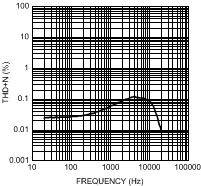VDD = 5 V POUT = 1.2 W RL = 4 Ω
Figure 3. THD+N vs Frequency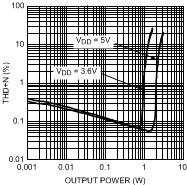f = 1 kHz RL = 4 Ω
Figure 5. THD+N vs Output Powerf = 1 kHz RL = 4 Ω
Figure 7. Power Dissipation vs Output Power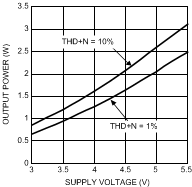f = 1 kHz RL = 4 Ω
Figure 9. Output Power vs Supply Voltage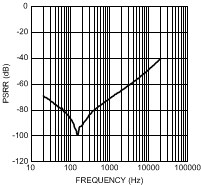VDD = 3.6 V VRIPPLE = 200 mVP-P RL = 8 Ω
Figure 11. PSRR vs Frequency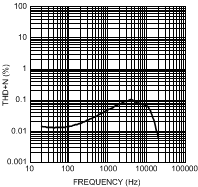VDD = 3.6 V POUT = 400 mW RL = 8 Ω
Figure 2. THD+N vs FrequencyVDD = 5 V POUT = 850 mW RL = 8 Ω
Figure 4. THD+N vs Frequency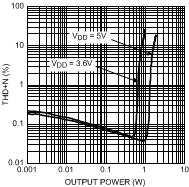f = 1 kHz RL = 8 Ω
Figure 6. THD+N vs Output Powerf = 1 kHz RL = 8 Ω
Figure 8. Power Dissipation vs Output Powerf = 1 kHz RL = 8 Ω
Figure 10. Output Power vs Supply Voltage